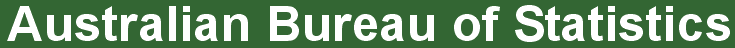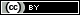ABS Home
 Statistical Language - Statistical Language GlossaryStatistical Language Glossary

A B C D E F G H I J K L M N O P Q R S T U V W X Y Z

A

Absolute frequency

The absolute frequency describes the number of times a particular value for a variable (data item) has been observed to occur.

Administrative data are collected as part of the day to day processes and record keeping of organisations.

See: Data Sources

B

Bar chart

A bar chart is a type of graph in which each column (plotted either vertically or horizontally) represents a categorical variable or a discrete ungrouped numeric variable.

C

Categorical variable

Categorical variables have values that describe a 'quality' or 'characteristic' of a data unit, like 'what type' or 'which category'.

Causation

Causation indicates that one event is the result of the occurrence of the other event; i.e. there is a causal relationship between the two events. This is also referred to as cause and effect.

Census (complete enumeration)

A census is a study of every unit, everyone or everything, in a population.

Classifications

Classifications are used to collect and organise information into categories with other similar pieces of information.

Class interval

A class interval is a range of data values. Each class interval has a lower and upper limit and contains all observations with values in that range. Class intervals cannot overlap with one another. For example 0 - 4, 5 - 8, 9 - 12.

Cohort

A cohort is a group of data units sharing a common experience or characteristic.

Comparability

Comparability is the ability to validly compare statistics that have been collected over time, or from different sources.

Confidence interval

A confidence interval is a range in which it is estimated the true population value lies.

Confidentiality

Confidentiality refers to the obligation of organisations that collect information to ensure that no person or organisation is likely to be identified from any data released.

See: Confidentiality

Continuous variable

A continuous variable is a numeric variable. Observations can take any value between a certain set of real numbers.

Correlation

Correlation is a statistical measure (expressed as a number) that describes the size and direction of a relationship between two or more variables.

Coverage

The coverage is the actual population of units within the scope of a data collection about which data can actually be collected. As it is not always possible to collect data from units in the population of interest, units may be in scope but not in coverage.

Cyclical effect

A cyclical effect is any regular fluctuation in daily, weekly, monthly or annual data.

See: Time Series Data

D

Data

Data are measurements or observations that are collected as a source of information.

See: What are Data?

Data item (or variable)

A data item is a characteristic (or attribute) of a data unit which is measured or counted, such as height, country of birth, or income.

See: What are Data?

Dataset

A dataset is a complete collection of all observations.

See: What are Data?

Data unit

A data unit is one entity (such as a person or business) in the population being studied, about which data are collected.

See: What are Data?

Data visualisation

Data visualisation involves the visual presentation of data to communicate the stories contained in the dataset.

Descriptive (or summary) statistics

Descriptive statistics summarise the raw data and allow data users to interpret a dataset more easily.

Discrete variable

A discrete variable is a numeric variable. Observations can take a value based on a count from a set of distinct whole values.

E

Error (Statistical error)

Statistical error describes the difference between a value obtained from a data collection process and the 'true' value for the population.

See: Types of Error

Estimate

An estimate is a value that is inferred for a population based on data collected from a sample of units from that population.

F

Flow series

A flow series is a series which is a measure of activity over a given period.

See: Time Series Data

Frequency

The frequency is the number of times a particular value for a variable (data item) has been observed to occur.

Frequency distribution

Frequency distributions are visual displays that organise and present frequency counts so that the information can be interpreted more easily.

H

Histogram

A histogram is a type of graph in which each column represents a numeric variable, in particular that which is continuous and/or grouped.

I

Index number

An index number is a ratio measuring the value of a data item at one time in relation to its value at a base period. Index numbers measure change without giving the actual numerical value of the data item.

Inferential statistics

Inferential statistics are used to infer conclusions about a population from a sample of that population.

Interquartile range (IQR)

The interquartile range (IQR) is the difference between the upper (Q3) and lower (Q1) quartiles, and describes the middle 50% of values when ordered from lowest to highest.

Irregular effect

An irregular effect is any movement that occurred at a specific point in time, but is unrelated to a season or cycle.

See: Time Series Data

M

Mean

The mean is the sum of the value of each observation in a dataset divided by the number of observations. This is also known as the arithmetic average.

Measures of central tendency (centre or central location)

A measure of central tendency (also referred to as measures of centre or central location) is a summary measure that attempts to describe a whole set of data with a single value that represents the middle or centre of its distribution.

Measures of shape

Measures of shape describe the distribution (or pattern) of the data within a dataset.

Measures of spread describe how similar or varied the set of observed values are for a particular variable (data item).

Median

The median is the middle value in distribution when the values are arranged in ascending or descending order.

Metadata is the information that defines and describes data.

Mode

The mode is the most commonly occurring value in a distribution.

N

Nominal variable

A nominal variable is a categorical variable. Observations can take a value that is not able to be organised in a logical sequence.

Non-random (non-probability) sample

In a non-random (or non-probability) sample some units of the population have no chance of selection, the selection is non-random, or the probability of their selection can not be determined.

Non-sampling error

Non-sampling error is caused by factors other than those related to sample selection.

See: Types of Error

Normal distribution

A normal distribution is a true symmetric distribution of observed values.

Numeric variable

Numeric variables have values that describe a measurable quantity as a number, like 'how many' or 'how much'.

O

Observation

An observation is an occurrence of a specific data item that is recorded about a data unit.

See: What are Data?

Ordinal variable

An ordinal variable is a categorical variable. Observations can take a value that can be logically ordered or ranked.

Original time series

An original time series shows the actual movements in the data over time.

See: Time Series Data

Outlier

Outliers are extreme, or atypical data value(s) that are notably different from the rest of the data.

P

Percentage

A percentage expresses a value for a variable in relation to a whole population as a fraction of one hundred.

Population

A population is any complete group with at least one characteristic in common.

Projection

A projection indicates what the future changes in a population would be if the assumptions about future trends actually occur.

Proportion

A proportion describes the share of one value for a variable in relation to a whole.

Q

Qualitative data

Qualitative data are measures of 'types' and may be represented by a name, symbol, or a number code.

Quantitative data

Quantitative data are measures of values or counts and are expressed as numbers.

Quartiles

Quartiles divide an ordered dataset into four equal parts, and refer to the values of the point between the quarters. A dataset may also be divided into quintiles (five equal parts) or deciles (ten equal parts).

R

Random (probability) sample

In a random (or probability) sample each unit in the population has a chance of being selected, and this probability can be accurately determined.

Range

The range is the difference between the smallest value and the largest value in a dataset.

Rate

A rate is a measurement of one value for a variable in relation to another measured quantity.

Ratio

A ratio compares the frequency of one value for a variable with another value for the variable.

Relative frequency

A relative frequency describes the number of times a particular value for a variable (data item) has been observed to occur in relation to the total number of values for that variable.

Relative standard error (RSE)

The relative standard error (RSE) is the standard error expressed as a proportion of an estimated value.

Respondent

A respondent provides data about oneself as a unit, or as a representative of another unit in a population.

See: Data Sources

S

Sample (partial enumeration)

A sample is a subset of units in a population, selected to represent all units in a population of interest.

Sampling error

Sampling error occurs solely as a result of using a sample from a population, rather than conducting a census (complete enumeration) of the population.

See: Types of Error

Scope

The scope is the set of units that comprise the population of interest (target population) about which data are being collected.

Seasonal effect

A seasonal effect is any variation in data due to calendar related effects which occur systematically at specific seasonal frequencies every year.

See: Time Series Data

A seasonally adjusted series involves estimating and removing the cyclical and seasonal effects from the original data.

See: Time Series Data

Skewness (skewed distribution)

Skewness is the tendency for the values to be more frequent around the high or low ends of the x-axis.

Standard deviation

The standard deviation measures the spread of the data around the mean.

Standard error (SE)

The standard error (SE) is a measure of the variation between any estimated population value that is based on a sample rather than true value for the population.

Statistical literacy

Statistical literacy refers to the knowledge and skills that enable data users and producers to understand, evaluate and communicate statistical data and information.

Statistical standard

A statistical standard is a set of rules used to standardise the way data are collected and statistics are produced.

Statistic

A statistic is a value that has been produced from a data collection, such as a summary measure, an estimate or projection. Statistical information is data that has been organised to serve a useful purpose.

Stock series

A stock series is a measure of certain attributes at a point in time and can be thought of as “stock takes”.

See: Time Series Data

Survey

A survey involves collecting information from every unit in the population (a census), or from a subset of units (a sample) from the population.

See: Data Sources

T

Time series

A time series is a collection of observations of well-defined data items obtained through repeated measurements over time.

See: Time Series Data

Trend series

A trend series is a seasonally adjusted series that has been further adjusted to remove irregular effects and 'smooth' out the series to show the overall 'trend' of the data over time.

See: Time Series Data

V

Variable (data item)

A variable is any characteristic, number, or quantity that can be measured or counted.

Variance

The variance measures the spread of the data around the mean.

 Privacy | Disclaimer | Feedback || ę Copyright| Sitemap| Online Security